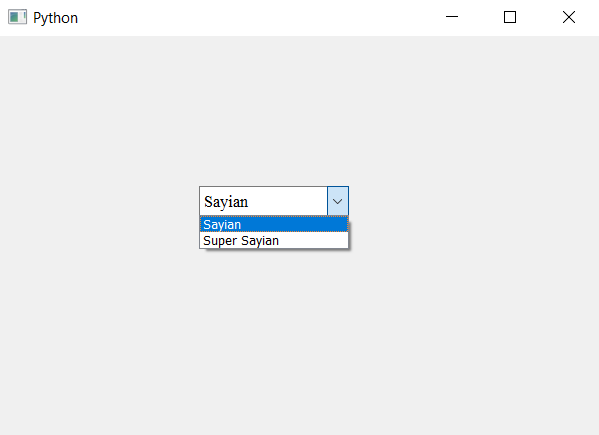# PyQt5 – Setting font to the item in line edit box of ComboBox

• Last Updated : 22 Apr, 2020

In this article we will see how we can change the size and font of the item present in the editable. If we use `setFont` method which takes `QFont` object as argument, using it with the check box object will change the font of all the items present in the list.

In order to change the font and size of only the item which is in the editable box, do the following –

1. Create a combo box
2. Make it editable combo box(optional)
3. Get the line edit(editable box) object from combo box
4. Change the font of the line edit using `setFont` method

Below is the implementation –

 `# importing libraries``from` `PyQt5.QtWidgets ``import` `*` `from` `PyQt5 ``import` `QtCore, QtGui``from` `PyQt5.QtGui ``import` `*` `from` `PyQt5.QtCore ``import` `*` `import` `sys`` ` ` ` `class` `Window(QMainWindow):`` ` `    ``def` `__init__(``self``):``        ``super``().__init__()`` ` `        ``# setting title``        ``self``.setWindowTitle(``"Python "``)`` ` `        ``# setting geometry``        ``self``.setGeometry(``100``, ``100``, ``600``, ``400``)`` ` `        ``# calling method``        ``self``.UiComponents()`` ` `        ``# showing all the widgets``        ``self``.show()`` ` `    ``# method for widgets``    ``def` `UiComponents(``self``):`` ` `        ``# creating a combo box widget``        ``self``.combo_box ``=` `QComboBox(``self``)`` ` `        ``# setting geometry of combo box``        ``self``.combo_box.setGeometry(``200``, ``150``, ``150``, ``30``)`` ` `        ``# geek list``        ``geek_list ``=` `[``"Sayian"``, ``"Super Sayian"``]`` ` `        ``# making it editable``        ``self``.combo_box.setEditable(``True``)`` ` `        ``# adding list of items to combo box``        ``self``.combo_box.addItems(geek_list)`` ` `        ``# font``        ``font ``=` `QFont(``'Times'``, ``10``)`` ` `        ``# getting the line edit of combo box``        ``line_edit ``=` `self``.combo_box.lineEdit()`` ` `        ``# setting line font to the line edit``        ``line_edit.setFont(font)`` ` `# create pyqt5 app``App ``=` `QApplication(sys.argv)`` ` `# create the instance of our Window``window ``=` `Window()`` ` `# start the app``sys.exit(App.``exec``())`

Output :My Personal Notes arrow_drop_up